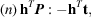International
Tables for
Crystallography
Volume B
Reciprocal space
Edited by U. Shmueli

International Tables for Crystallography (2010). Vol. B, ch. 1.4, pp. 120-121   | 1 | 2 |

## Section 1.4.4.4. Symmetry in Fourier space

U. Shmuelia

#### 1.4.4.4. Symmetry in Fourier space

| top | pdf |

As shown below, Table A1.4.4.1can also be regarded as a collection of the general equivalent positions of the symmetry groups of Fourier space, in the sense of the treatment by Bienenstock & Ewald (1962). This interpretation of the table is, however, restricted to the underlying periodic function being real and positive (see the latter reference). The symmetry formalism can be treated with the aid of the originalmatrix notation, but it appears that a concise Seitz-type notation suits better the present introductory interpretation.

The symmetry dependence of the fundamental relationship (1.4.2.5)is given by a table entry of the form:where the phase shift is given in units of 2π, and the structure-dependent phaseis omitted. Defining a combination law analogous to Seitz's product of two operators of affine transformation:where R is amatrix,is a row vector, r is a column vector and b is a scalar, we can write the general form of a table entry aswhere δ is a constant phase shift which we take as zero. The positionsandare now related by the operationvia the combination law (1.4.4.9), which is a shorthand transcription of thematrix notation of Bienenstock & Ewald (1962), with the appropriate sign of t.

Let us evaluate the result of a successive application of two such operators, sayandto the reference positionin Fourier space:and perform an inverse operation:These equations confirm the validity of the shorthand notation (1.4.4.9)and illustrate the group nature of the operatorsin the present context.

Following Bienenstock & Ewald, the operatorsare symmetry operators that act on the positionsin Fourier space, provided they satisfy the following requirements: (i) the application of such an operator leaves the magnitude of the (generally) complex Fourier coefficient unchanged, and (ii) after g successive applications of an operator, where g is the order of its rotation part, the phase remains unchanged up to a shift by an integer multiple of 2π (a trivial phase shift, corresponding to a translation by a lattice vector in direct space).

If our function is the electron density in the crystal, the first requirement is obviously satisfied since, where F is the structure factor [cf. equation (1.4.2.4)]. In order to make use of the second requirement in deriving permissible symmetry operators on Fourier space, all the relevant transformations, i.e. those which have rotation operators of the orders 1, 2, 3, 4 and 6, must be individually examined. A comprehensive example, covering most of the tetragonal system, can be found in Bienenstock & Ewald (1962).

It is of interest to illustrate the above process for a simple particular instance. Consider an operation, the rotation part of which involves a mirror plane, and assume that it is associated with the monoclinic system, in the second setting (unique axis b). We denote the operator bywhere, and the permissible values of u, v and w are to be determined. The operation is of order 2, and according to requirement (ii) above we have to evaluatewhereis the matrix representing the operation of reflection and I is the unit matrix. Forto be an admissible symmetry operator, the phase-shift part of (1.4.4.13), i.e., must be an integer (multiple of 2π). The smallest non-negative values of u and w which satisfy this are the pairs:,and,and, and. We have thus obtained four symmetry operators in Fourier space, which are identical (except for the sign of their translational parts) to those of the direct-space monoclinic mirror and glide-plane operations. The fact that the component v cancels out simply means that an arbitrary component of the phase shift can be added along theaxis; this is concurrent with arbitrary direct-space translations that appear in the characterization of individual types of space-group operations [see e.g. Koch & Fischer (2005)].

Each of the 230 space groups, which leaves invariant a (real and non-negative) function with the periodicity of the crystal, thus has its counterpart which determines the symmetry of the Fourier expansion coefficients of this function, with equivalent positions given in Table A1.4.4.1.

### References

Bienenstock, A. & Ewald, P. P. (1962). Symmetry of Fourier space. Acta Cryst. 15, 1253–1261.
Koch, E. & Fischer, W. (2005). In International Tables for Crystallography, Vol. A, Part 11, Symmetry operations. Heidelberg: Springer.